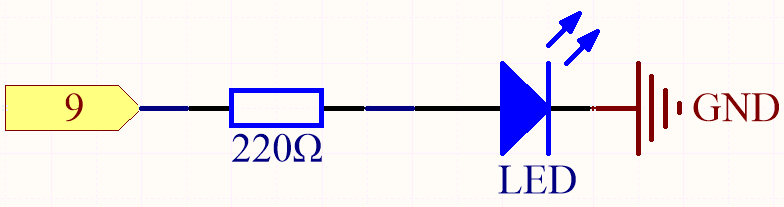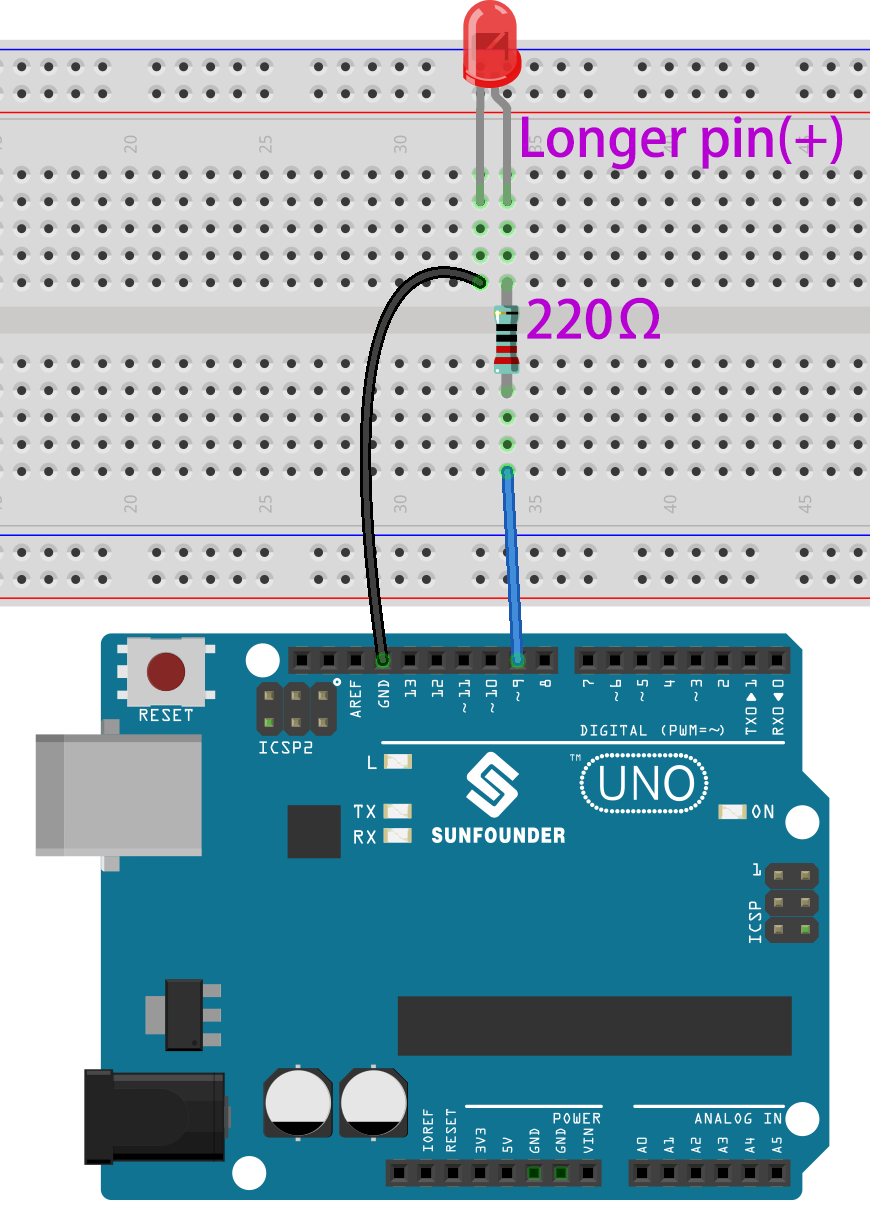# 1.1 Hello, LED!¶

Just as printing “Hello, world!” is the first step in learning to program, using a program to drive an LED is the traditional introduction to learning physical programming.

Required Components

In this project, we need the following components.

Name

ITEMS IN THIS KIT

3 in 1 Starter Kit

380+

COMPONENT INTRODUCTION

SunFounder R3 Board

Jumper Wires

Resistor

LED

SchematicThe principle of this circuit is simple and the current direction is shown in the figure. When pin 9 outputs high level(5V), the LED will light up after the 220ohm current limiting resistor. When pin 9 outputs low level (0v), the LED will turn off.

WiringCode

Note

• You can open the file `1.1.hello_led.ino` under the path of `3in1-kit\basic_project\1.1.hello_led`.

• Or copy this code into Arduino IDE.

• Or upload the code through the Arduino Web Editor.

After the code is uploaded successfully, you will see the LED blinking.

How it works?

Here, we connect the LED to the digital pin 9, so we need to declare an int variable called ledpin at the beginning of the program and assign a value of 9.

```const int ledPin = 9;
```

Now, initialize the pin in the `setup()` function, where you need to initialize the pin to `OUTPUT` mode.

```void setup() {
pinMode(ledPin, OUTPUT);
}
```

In `loop()`, `digitalWrite()` is used to provide 5V high level signal for ledpin, which will cause voltage difference between LED pins and light LED up.

```digitalWrite(ledPin, HIGH);
```

If the level signal is changed to LOW, the ledPin’s signal will be returned to 0 V to turn LED off.

```digitalWrite(ledPin, LOW);
```

An interval between on and off is required to allow people to see the change, so we use a `delay(1000)` code to let the controller do nothing for 1000 ms.

```delay(1000);
```